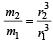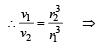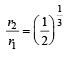Courses

# 28 Year NEET Questions: Nuclei- 3

## 30 Questions MCQ Test Physics 28 Years Past year papers for NEET/AIPMT Class 12 | 28 Year NEET Questions: Nuclei- 3

Description
This mock test of 28 Year NEET Questions: Nuclei- 3 for NEET helps you for every NEET entrance exam. This contains 30 Multiple Choice Questions for NEET 28 Year NEET Questions: Nuclei- 3 (mcq) to study with solutions a complete question bank. The solved questions answers in this 28 Year NEET Questions: Nuclei- 3 quiz give you a good mix of easy questions and tough questions. NEET students definitely take this 28 Year NEET Questions: Nuclei- 3 exercise for a better result in the exam. You can find other 28 Year NEET Questions: Nuclei- 3 extra questions, long questions & short questions for NEET on EduRev as well by searching above.
QUESTION: 1

### A radioactive sample with a half life of 1 monthhas the label : ‘Activity = 2 micro curies on 1–8–1991. What would be its activity two monthsearlier ? 

Solution:

In two half lives, the activity becomes one fourth.
Activity on 1–8–91 was 2 micro–curie
∴ Activity before two months, 4 × 2 micro-curie = 8 micro curie

QUESTION: 2

### The nucleus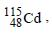after two successive β – decay will give

Solution:

Two successive β decay increase the charge number by 2. Therefore, (d) is correct.

QUESTION: 3

### A radioactive element has half life period 800 years. After 6400 years what amount will remain? 

Solution:

No. of half lives,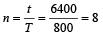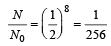QUESTION: 4

An element A decays into element C by a two step process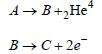then            

Solution:

From eqn. (ii), B has 2 units of charge more than C.
From eqn. (i), A loses 2 units of charge by emission of alpha particle. Hence, A and C are isotopes as their charge numbers are same.

QUESTION: 5

Ultraviolet radiations of 6.2 eV falls on analuminium surface. K.E. of fastest electronemitted is (work function = 4.2 eV) 

Solution:

K.E. of fastest electron = E – W0 = 6.2 – 4.2 = 2.0 eV
= 2 × 1.6 × 10–19 = 3.2 × 10– 19 J

QUESTION: 6

Curie is a unit of      

Solution:

Curie is a unit of radioactivity.

QUESTION: 7

The average binding energy of a nucleon insidean atomic nucleus is about      

Solution:

Average B.E./nucleon in nuclei is of the order of 8 MeV.

QUESTION: 8

The nuclei 6C13 and 7N14 can be described as              

Solution:

As 6C13 and 7N14 have same no. of neutrons (13 – 6 =7 for C and 14 – 7 =7 for N), so they are isotones.

QUESTION: 9

Which of the following statements is true fornuclear forces?  

Solution:

Nuclear forces are short range attractive forces which balance the repulsive forces between the protons inside the nucleus.

QUESTION: 10

The ratio of the radii of the nuclei 13Al27 and 52Te125 is approximately 

Solution: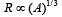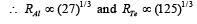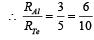QUESTION: 11

The nucleus 126C absorbs an energetic neutronand emits a beta particle (β). The resultingnucleus is 

Solution: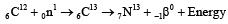QUESTION: 12

The constituents of atomic nuclei are believedto be  

Solution:

Nucleus contains only neutrons and protons.

QUESTION: 13

The half life of radium is 1600 years. The fractionof a sample of radium that would remain after6400 years     

Solution: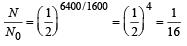QUESTION: 14

An electron with (rest mass m0) moves with a speed of 0.8 c. Its mass when it moves with this speed is    

Solution: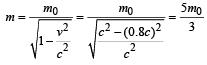QUESTION: 15

In the nucleus of 11Na23, the number of protons,neutrons and electrons are 

Solution:

Z = 11 i.e., number of protons = 11, A = 23
∴ Number of neutrons = A – Z = 12
Number of electron = 0 (No electron in nucleus) Therefore 11, 12, 0.

QUESTION: 16

If the nuclear force between two protons, two neutrons and between proton and neutron is denoted by Fpp, Fnn and Fpn respectively, then 

Solution:

Nuclear force is the same between any two nucleons.

QUESTION: 17

Solar energy is due to 

Solution:

Fusion reaction.

QUESTION: 18

The energy equivalent of one atomic mass unitis 

Solution:

1 a.m.u = 931 MeV

QUESTION: 19

The mass of α-particle is 

Solution:

α-particle = 2He4 . It contains 2p and 2n.
As some mass is converted into B.E., therefore, mass of α particle is slightly less than the sum of the masses of 2p and 2n.

QUESTION: 20

The mass density of a nucleus varies with massnumber A as 

Solution:

The nuclear radius r varies with mass number A according to the relation
r ∝ A1/3 or A ∝ r3

Now,  density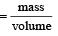Further, mass ∝ A and volume ∝ r3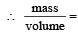constant

QUESTION: 21

In a given reaction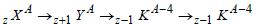Solution:

Increase of charge number. by 1 indicates β emission. Decrease of mass number by 4 and charge number by 2 indicates α emission.
No change of mass number and charge number indicates γ-emission.

QUESTION: 22

The binding energy per nucleon is maximum in case of

Solution:

From B.E. curve, the curve reaches peak for
26 Fe56

QUESTION: 23

Energy released in the fission of a single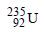nucleus is 200 MeV. The fission rate of afilled reactor operating at a power level of 5 W is            

Solution:

Fission rate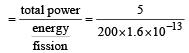= 1.56 × 1011 s–1

QUESTION: 24

If the binding energy per nucleon in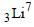and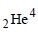nuclei are respectively 5.60 MeV and 7.06 MeV, then the energy of proton in the reaction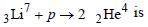Solution:

Applying principle of energy conservation, Energy of proton = total B.E. of 2α– energy of Li7
= 8 × 7.06 – 7 × 5.6
= 56.48 – 39.2 = 17.28 MeV

QUESTION: 25

Heavy water is used as a moderator in a nuclearreactor. The function of the moderator is

Solution:

Moderator slows down the neutrons to thermal energies.

QUESTION: 26

The mass number of He is 4 and that for sulphur is 32. The radius of sulphur nuclei is larger than that of helium by [1994, 1995]

Solution: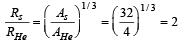QUESTION: 27

The nature of ions knocked out from hotsurfaces is 

Solution:

Ion is an atom or a group of atoms that has lost or gained electrons having negative or positive charge respectively. Thus, the ions knocked out from hot surfaces is electrons.

QUESTION: 28

What is the respective number of α and β particles emitted in the following radioactive decay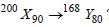?    

Solution: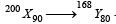We know that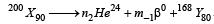Therefore, in this process,

200 = 4n + 168 or  n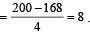Also, 90 = 2n – m + 80
or,  m = 2n + 80 – 90 = (2 × 8 + 80 – 90) = 6.
Thus,  respective number of α and β-particles will be 8 and 6.

QUESTION: 29

The count rate of a Geiger Muller counter for theradiation of a radioactive material of half-life 30minutes decreases to 5 sec–1 after 2 hours. Theinitial count rate was 

Solution:

Half-life = 30 minutes; Rate of decrease (N) = 5 per second and total time = 2 hours = 120 minutes. Relation for initial and final count rate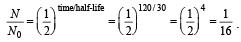Therefore, N0 = 16 × N = 16 × 5 = 80 s–1.

QUESTION: 30

A nucleus ruptures into two nuclear parts, whichhave their velocity ratio equal to 2:1. What willbe the ratio of their nuclear size (nuclear radius)?        

Solution:

Applying law of conservation of momentum,
m1v1 = m2v2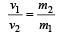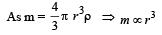Hence,